# Is <11 faster than <=10? - page 2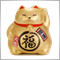1436

`The layman's explanation is this...`

Never ever discuss with layman ... they are way to smart to argue with, you'll never make your point.

(excuse me for feeding trolls)

;)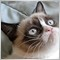919

Are you sure MQL uses that instructions? It's all in theory as I have said. No one among us knows what's inside the compiler. Your theory is as good as mine.

Thats exactly why thread started1370

Are you sure MQL uses that instructions? It's all in theory as I have said. No one among us knows what's inside the compiler. Your theory is as good as mine.

:
Thats exactly why thread started

Sorry to say that, but it's not a matter of theory but of facts. The answer has been given.

The facts are:
1. compilers turn codes into machine code.
2. in the machine code language, those 2 comparisons can be written in one instruction.
3. it's true that we don't know if the compiler actually uses this instruction, but there is no reason to believe that it's not. comparison is just a trivial operation in assembly and surely for compilers and compiler writers. MQL5 compiler is a good performance compiler, you will not expect something so trivial to be written as 2 operations for <= and one operation for < when the target language has a better twice as fast option.
4. The < and <= are translated to a set of machine code instructions. Even if we assume that those are 2 different sets of instructions, they come to a point were the only difference between the 2 sets is that comparison - which means that they are the same. The first set + the < machine instruction vs. the second set + the <= machine instruction. This must be the only difference between the 2 sets - one machine instruction against the other - and that means they are the same.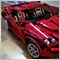1105

I did this simple test:

```   ulong iStart = 0, iStop = 0;
int iVar1 = 10, iVar2 = 15;
bool bResult;

iStart = GetMicrosecondCount();
for (int i=0; i<INT_MAX; i++)
bResult = iVar1<iVar2;
iStop = GetMicrosecondCount();
Print ("Microseconds elapsed (<) = ", iStop-iStart);

iStart = GetMicrosecondCount();
for (int i=0; i<INT_MAX; i++)
bResult = iVar1<=iVar2;
iStop = GetMicrosecondCount();
Print ("Microseconds elapsed (<=) = ", iStop-iStart);
```

And I gathered these results:

Test Run 1 Run 2 Run 3 Run 4 Run 5
< 5573237 5538121 5640963 5604254 5566550
<= 5618423 5582935 5671609 5623322 5600941

Clearly, < is faster than <=, although the difference is negligible.1370

It clearly does not say what you claim (average difference is 0.62 percent).

My tests show the opposite: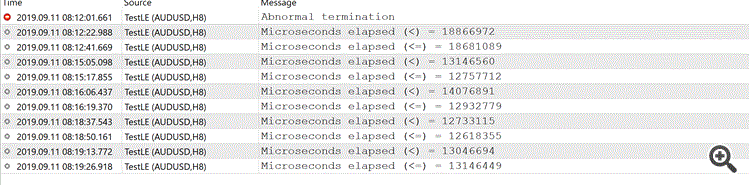1105

Amir Yacoby:

It clearly does not say what you claim (average difference is 0.62 percent).

My tests show the opposite:

🤣 Not that clear after all.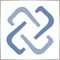1390

Exactly the same in MQL4 and MQL5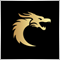23741

Seng Joo Thio:

I did this simple test:

And I gathered these results:

Test Run 1 Run 2 Run 3 Run 4 Run 5
< 5573237 5538121 5640963 5604254 5566550
<= 5618423 5582935 5671609 5623322 5600941

Clearly, < is faster than <=, although the difference is negligible.

That is expected. The line "if(iVar1<iVar2)" consists of 14 characters. While the line "if(iVar1<=iVar2)" has 15 characters. In a compiler, before translation, each character is fetch with multiple loops. Each loop uses microseconds of execution. Then another loop is used for the first question that checks "<", if it's false it will check for the next condition which is "=" of the "<=" that consumes another microseconds of execution.  The assembly language jump instructions is not a direct translation of the "if" condition of MQL. Those jump instructions are used as a part of implementation of the process of the translation.

Try to make a third test for "<=". Make iVar1=15 also. That would make iVar1=iVar2. If it makes it even slower than the first two above, it means the MQL compiler is using the "2 questions" method I'm talking about above.1370

That is expected. The line "if(iVar1<iVar2)" consists of 14 characters. While the line "if(iVar1<=iVar2)" has 15 characters. In a compiler, before translation, each character is fetch with multiple loops. Each loop uses microseconds of execution. Then another loop is used for the first question that checks "<", if it's false it will check for the next condition which is "=" of the "<=" that consumes another microseconds of execution.  The assembly language jump instructions is not a direct translation of the "if" condition of MQL. Those jump instructions are used as a part of implementation of the process of the translation.

Try to make a third test for "<=". Make iVar1=15 also. That would make iVar1=iVar2. If it makes it even slower than the first two above, it means the MQL compiler is using the "2 questions" method I'm talking about above.

You seem to confuse execution with  parsing. Parsing is done before execution in compilers, it won't affect the execution time which starts only after all parsing is finished and the assembly program is ready and only then it is run. As also confirmed by Ilyas 2 posts above, this is the answer.38835